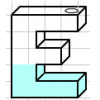#### You may also like### Speedy Sidney

Two trains set off at the same time from each end of a single straight railway line. A very fast bee starts off in front of the first train and flies continuously back and forth between the two trains. How far does Sidney fly before he is squashed between the two trains?### Air Nets

Can you visualise whether these nets fold up into 3D shapes? Watch the videos each time to see if you were correct.### Maths Filler

Imagine different shaped vessels being filled. Can you work out what the graphs of the water level should look like?

# Temperature

### Why do this problem?

This problem offers an opportunity to combine skills from mathematics and science. It can be solved numerically, algebraically or graphically, so can offer a useful opportunity for discussing the merits of different methods.

### Possible approach

This printable worksheet may be useful: Temperature.

Introduce the boiling and freezing point of water in Celsius and Fahrenheit.

The freezing point of water is $0^\circ C$ and $32^\circ F$.
The boiling point of water is $100^\circ C$ and $212^\circ F$.

"What other information can you deduce from these temperature facts?"
Give the class some time to discuss in pairs, then bring the class together to collect ideas on the board. Possible responses might be:
"$50^\circ C = 122^\circ F$ because it's halfway between."
"$200^\circ C = 392^\circ F$ because it's another $180^\circ F$."
"A temperature increase of $100^\circ C$ is the same as a temperature increase of $180^\circ F$."

Again, give the class some time to discuss in pairs, and then collect ideas once more.

"Is there a temperature where the reading in Celsius is the same as the reading in Fahrenheit?"

Give the class plenty of time to tackle this problem. As they work, circulate and look out for the different approaches they are using. Most students are likely to use a numerical approach. If some students use algebraic or graphical methods, ask them to share their approaches with the rest of the class.

If nobody uses algebra or graphs, ask the class to consider first how a graph might help:
"Can you represent the original information graphically in a way that could have helped you to solve the problem?"

The graphical method can then lead on to a discussion of the algebraic representation of the straight line graph and hence algebraic methods of solution.

Take time to discuss the merits of the different methods and then challenge students to show how to use each solution method to solve problems such as:
"Is there a temperature at which the Fahrenheit reading is 20 degrees higher than the Celsius reading?"
"Is there a temperature at which the Celsius reading is 20 degrees higher than the Fahrenheit reading?"

### Key questions

Does every method give the same answer?
What are the merits of the different methods?

### Possible extension

See the extension challenge introducing the Kelvin scale of temperature in the problem.

### Possible support

Spend lots of time discussing how to deduce information from the initial temperature facts given. Perhaps it would help students to suggest new values if the information is presented in a table.